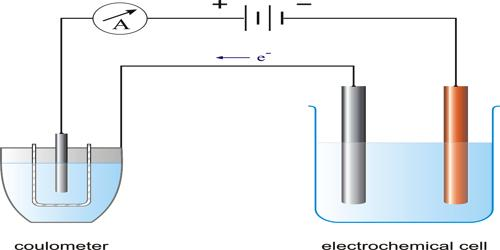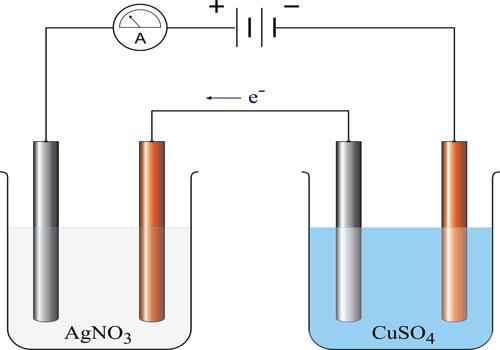Chemistry

# Verification of the First Law of FaradayVerification of the First Law of Faraday

Faraday’s first law may be verified by measuring the quantity of electricity flowing through an electrical circuit. The apparatus used for the purpose is known as a voltammeter, or better a coulometer (Figure) because the same apparatus can be used to measure the quantity of electricity passed. In this apparatus the amount of the metal deposited on a clean electrode by passing a known current for a definite time through a solution of a salt of the metal is determined.If AgNO3 solution is used as the electrolyte metallic Ag will be deposited on the cathode, generally a platinum basin containing the silver nitrate solution. The deposit is washed with water; dried and weighed. The mass of the metal deposited can be accurately determined by weighing the electrode before and after passage of the current. If a current ‘I’ ampere flows for t seconds, the quantity of electricity passed is I x t = Q coulombs. The mass of metal deposited on passing different quantities of electricity can be determined. The results shows, that the mass of metal deposited is proportional to Q, the quantity of electricity passed.